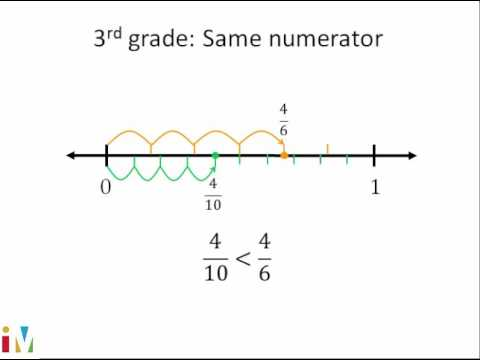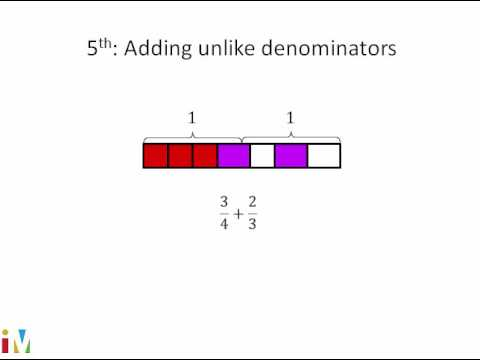Video

# Subtracting fractions with like denominators (Full video)

Description: Sal subtracts 8/18 - 5/18. We're asked to subtract and simplify the answer, and we have 8/18 minus 5/18. So in this case, it is 8 minus 5, and this will be equal to 3 over 18, which is the answer, but it's not completely simplified, because both 3 and 18 are divisible by 3. And just to see this visually, let me draw 18 parts.

### Other videos you might be interested in### Comparing Fractions

#### Illustrative Mathematics